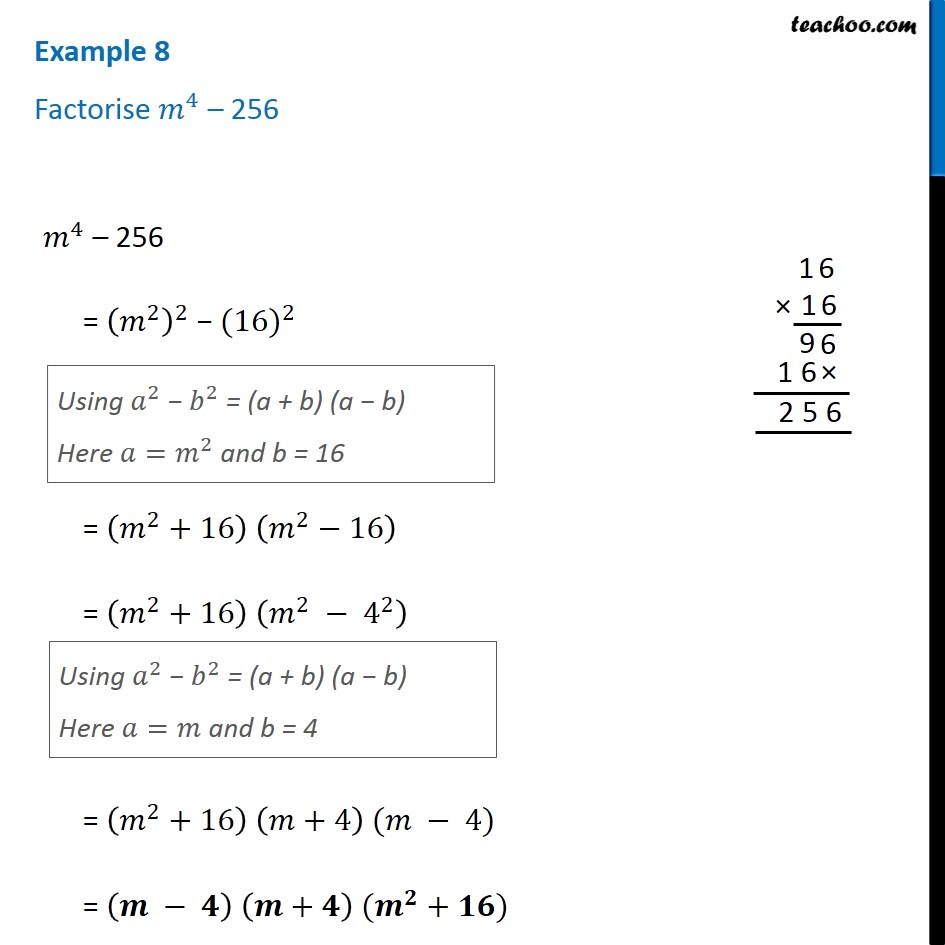1. Chapter 14 Class 8 Factorisation
2. Serial order wise
3. Examples

Transcript

Example 8 Factorise 𝑚^4 – 256 𝑚^4 – 256 = (𝑚^2 )^2 − 〖(16)〗^2 Using 𝑎^2 − 𝑏^2 = (a + b) (a − b) Here 𝑎=𝑚^2 and b = 16 = (𝑚^2+16) (𝑚^2−16) = (𝑚^2+16) (𝑚^2 − 4^2 ) Using 𝑎^2 − 𝑏^2 = (a + b) (a − b) Here 𝑎=𝑚 and b = 4 = (𝑚^2+16) (𝑚+4) (𝑚 − 4) = (𝒎 − 𝟒) (𝒎+𝟒) (𝒎^𝟐+𝟏𝟔)

Examples